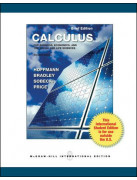

This course aims to teach students the basics of Algebra:

1.Operations with fractions and ratio / proportions as well as operations with algebraic expressions.

2. Factoring polynomials, details of linear equations, linear and absolute value inequalities.

3. Functions (lines and linear functions, limits, graphs and continuity).Contact: Jamel Chafra at chafra@bilkent.edu.tr